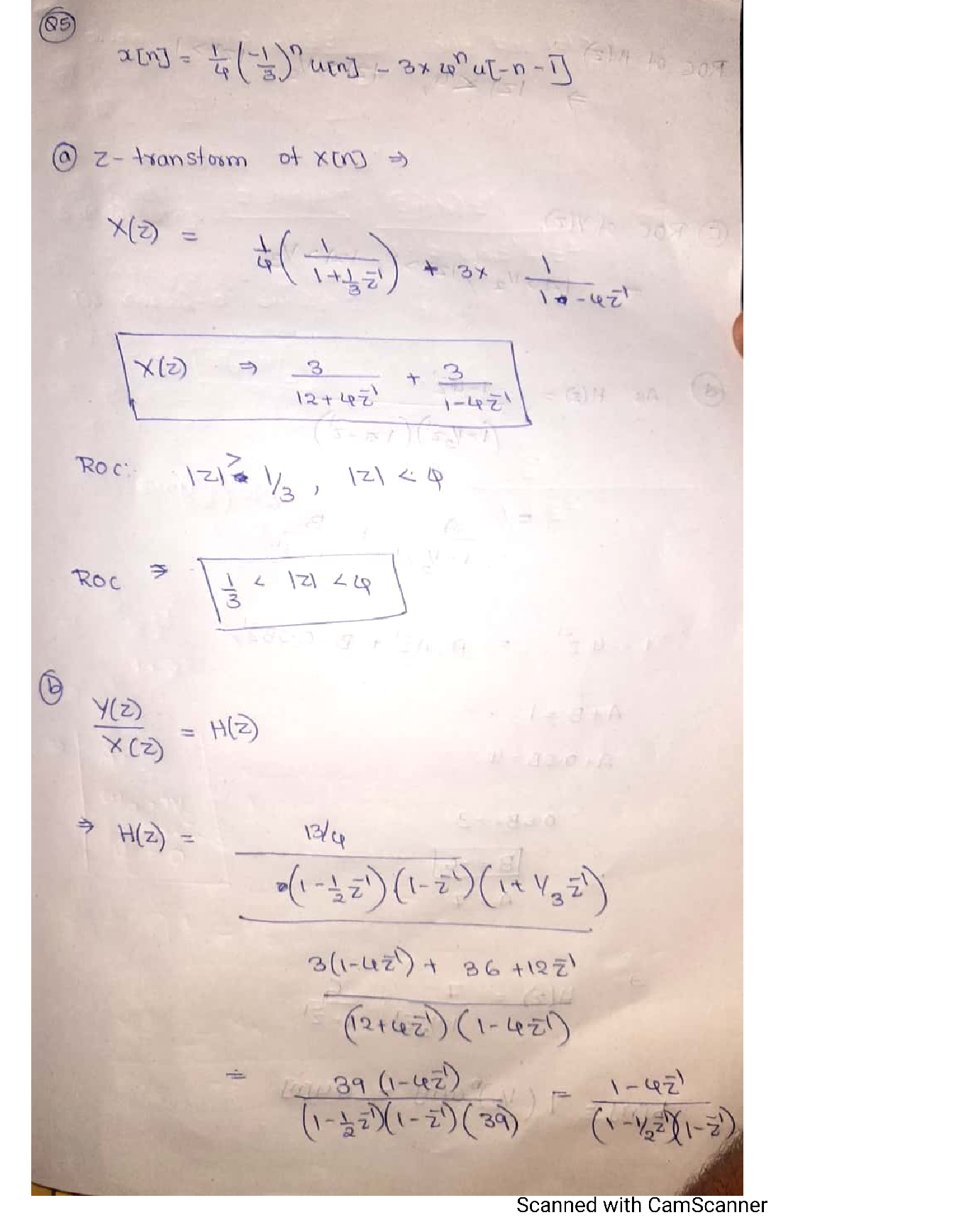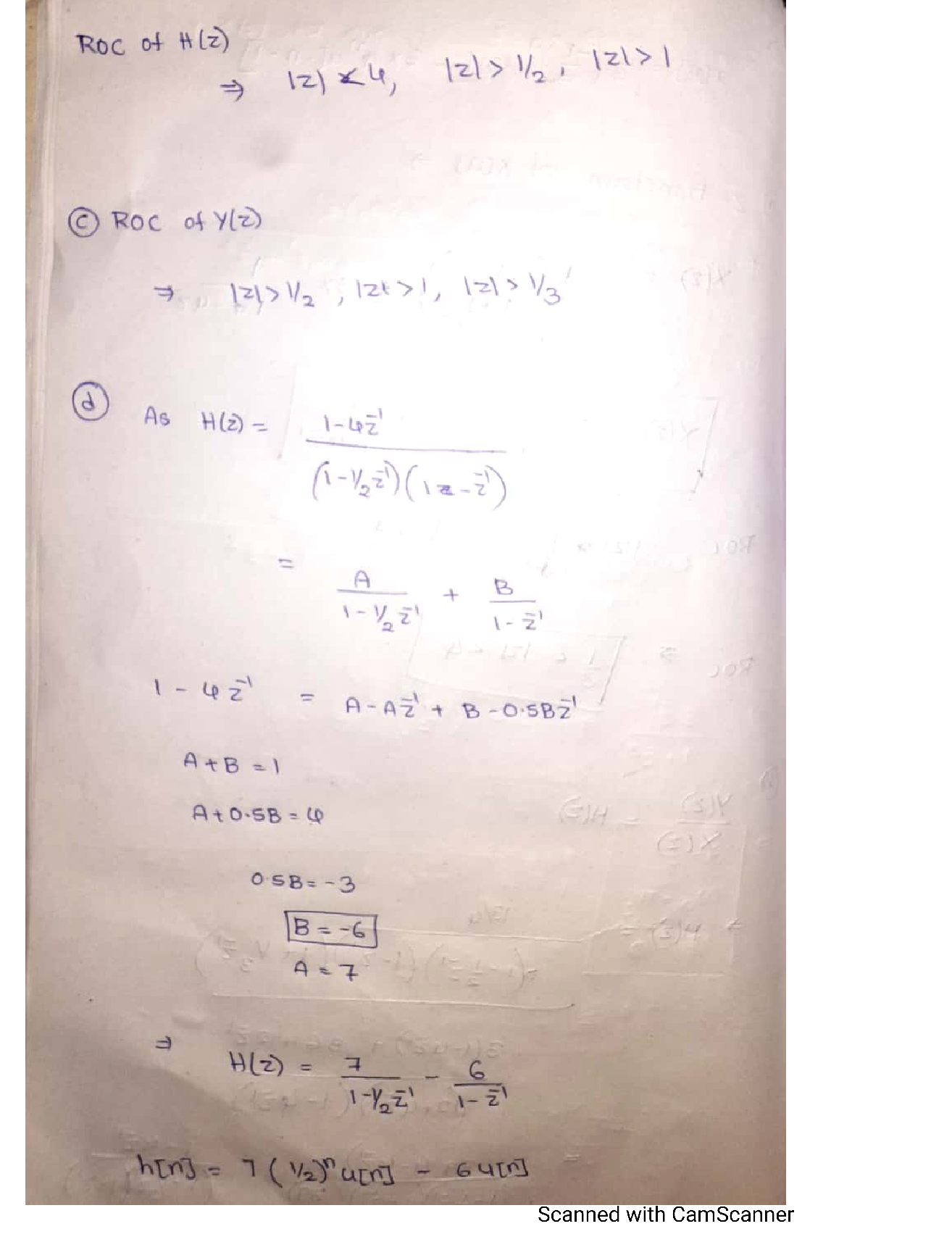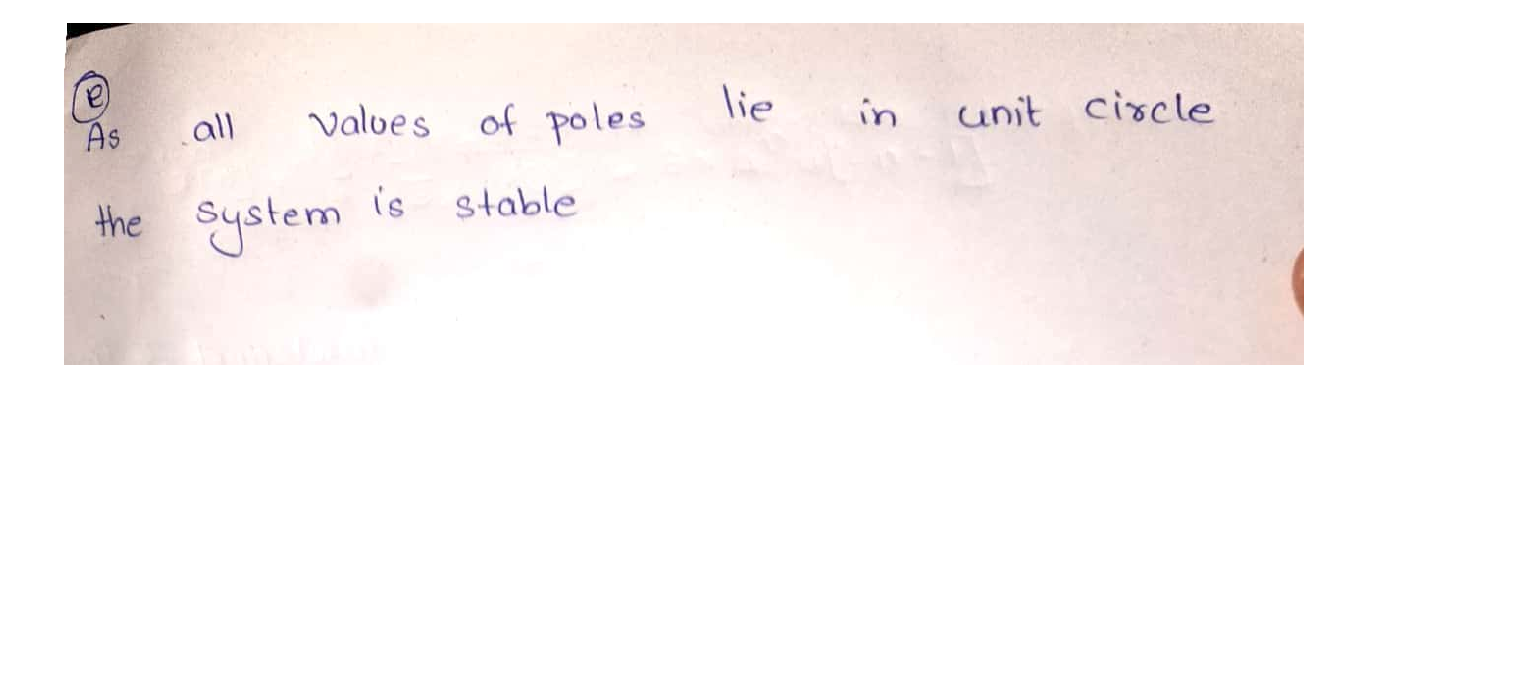Question

Signal Processing

\text { Suppose that the input to a causal LTI system is }

x[n]=\frac{1}{4}\left(-\frac{1}{3}\right)^{n} u[n]-3 \times 4^{n} u[-n-1]

and the z-transform of the output y[n] is

Y(z)=\frac{13 / 4}{\left(1-\frac{1}{2} z^{-1}\right)\left(1-z^{-1}\right)\left(1+\frac{1}{3} z^{-1}\right)}

(a) Find the z-transform of a[n] and its ROC.

b) Find the transfer function H(z) and its ROC.

:) Determine the ROC of Y(z).

(d) Find the impulse response of the system.

=) Is the system stable?Verified### Question 44811Signal Processing

Question 1. What is the output voltage Vout when PA2 is high, PA1 is high, and PAO is low? Assume VoH is3.3V and VoL = 0V.
In terms of a DAC define resolution, range, precision, monotonic.
How do you extend the 4-bit binary-weighted DAC to 6-bits?
. Design a 6-bit R-2R DAC.
Write C code that increments a variable, but forces it to a range of 0 to 31 (0,1,2,3, ... 29,30,31,0,1,2,..)

### Question 44553Signal Processing

, what is the lowest frequency at which good coupling exists?

### Question 44314Signal Processing

The discrete-circuit common-emitter amplifier in figure, the transistor is operating at a dc collector current of 1 mA and has B = 120, fr =1 GHz, and Cu = 0.5 pF.
(1)Find the midband gain Am
(ii)Calculate the low frequency FL
(ii)Calculate the low frequency FH

### Question 43972Signal Processing

\text { For the common-source amplifier in the Figure, } g_{m}=2 \frac{\mathrm{mA}}{V} \text {, and } C_{g s}=20 \mathrm{fF} \text { and } C_{g d}=5 \mathrm{fF} \text {. }
Draw the small signal equivalent circuit at mid-band and find the mid-band gain Am
Draw the small-signal equivalent circuit at low frequency and calculate FL.
Draw the small-signal equivalent circuit at high frequency and calculate FH.

### Question 40782Signal Processing

Problem Statement-A common emitter amplifiers shown has the following design parameters:BJT intrinsic parameters-
\beta_{\mathrm{f}}=80, r_{\pi}=1600 \Omega, C_{\pi}=10 \mathrm{pF}, C_{\mu}=10 \mathrm{pF}
External resistors are-
R_{S}=200 \Omega, R_{1}=7000 \Omega, R_{2}=4500 \Omega, R_{E}=300 \Omega, R_{C}=5 k \Omega, R_{L}=5 k \Omega
External capacitors
C_{1}=10 \mu F, C_{2}=2 \mu F, C_{E}=100 \mu F, C_{x}=10 \mathrm{pF}
Use short-circuit zero value single time-constant method to calculate
a)Midband gain vo/vs
b)Low frequency poles due to the three capacitors C1, C2, and CE
c)An estimate of the lower 3-dB frequency, fL
d)The high frequency poles
e)An estimate of the 3-dB frequency, fH

### Question 40270Signal Processing

(50 pts) Design a compensator for the system below to provide a closed-loop response thatsatisfies the following requirements:
\text { - } e_{5 s}=0 \text { for a constant reference }
-9005=5 \%
-t_{5} \approx 1.2 \mathrm{sec}(\pm 1 \%)

### Question 40269Signal Processing

Angle of Departure
(20 pts) Draw the root locus for the following system on the axes below. Show all calculations onthe following page for:
b. Gain value at imaginary axis crossings
C. Location of imaginary axis crossings

### Question 40268Signal Processing

s) For the following system, determine the steady-state error to a ramp input, r(t)2.5t.

### Question 40267Signal Processing

6. (10 pts) The open-loop Bode plot below is for a system with no open-loop right-half-plane poles.What is the system's phase margin (Give a numeric value and indicate on the plot)? What is the approximate overshoot of the closed-loop step response?

### Question 40266Signal Processing

(10 pts) The steady-state.response of a stable system.to a sinusoidal input function will, itself, bea sinusoid of the same frequency as the input function, with the only difference being the ratio of input to output amplitude, and a phase shift between the input and output sinusoids. Using the transfer function for the system (T(s) relating the input, u, to the output, y), how can you mathematically determine the amplitude ratio and the phase shift for a given input sinusoidal frequency, w?

### Submit query